PH 212

Oscillations• Simple harmonic motion
• Oscillator vs. wave
• Simple harmonic oscillators
• Mass on a spring
• Pendulum
• Physical pendulum
• Energy
• Damping
• Driven oscillations
• Resonance

Simple harmonic motion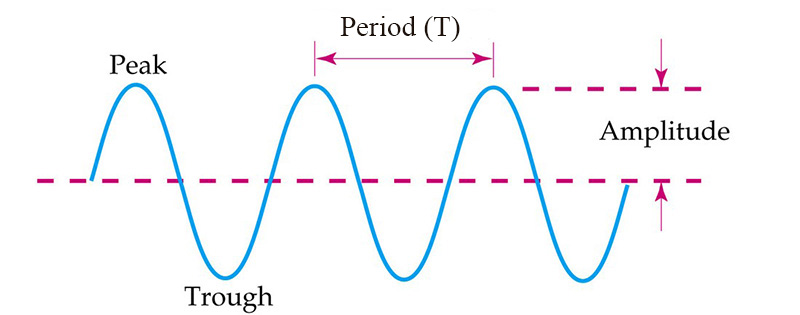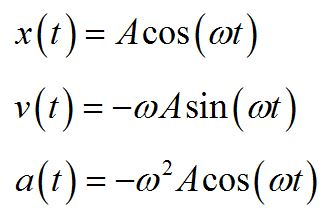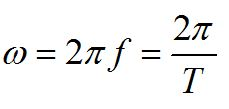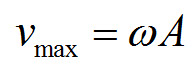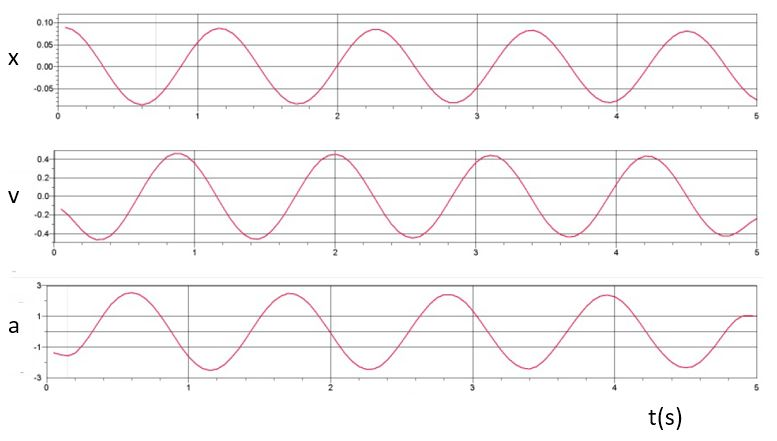An undamped mass oscillating on a spring produces a sine wave. Note that a maximum or minimum value of position corresponds with a zero in velocity. Acceleration is not constant, but varies out of phase with position vs. time.A rotating object can also be considered a simple harmonic oscillator. Its projection produces a sine wave.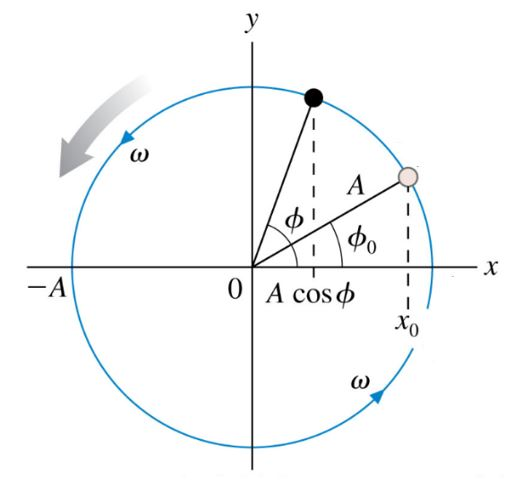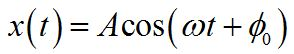The phase is the argument of the sine function. The phase constant provides initial conditions for the oscillation, when t = 0.

Simple harmonic oscillator: mass on a spring

© 2013 Pearson Education, Inc